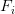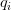# SciCast Accuracy Calculations

We have started adding “Accuracy” numbers to emails. For example:

• Your average accuracy on this question was 83.
• SciCast’s average accuracy on this question was 90.

What does that mean?  The short answer is that it’s a transform of the familiar Brier score, which we have mentioned in several blog posts.  Where the Brier measures your error (low is good), Accuracy measures your success (high is good). This is more intuitive … except when it’s not.

The Accuracy is a measure of the quality of your individual trades or estimates: how close did you get, on average, to the right answer?  Accuracy is different from market score.  A high market score does not necessarily imply good accuracy, and vice versa.

• The market pays you for information: 100 points per bit.  You can earn thousands of points by repeatedly moving the forecast 1% → 2% on an event that happens.  But your accuracy is terrible — far worse than flipping a coin.
• Accuracy “pays” for putting high probability on the right answer. You get nearly perfect accuracy by moving 98% → 99% just once on an event that happens. But you gain a measly 1.5 market points.

Stereotypical value traders (subject matter experts) have high accuracy but low market score.  Stereotypical technical traders have a high market score with a low accuracy.

# Simple Accuracy

We want an accuracy score with these properties:

• It is 100 for putting all your probability on the right answer.
• It is 0 for the uniform distribution.
• It is negative for doing worse than uniform.
• It is bounded.
• It works for mixture resolutions as well.
• Therefore it works for Scaled questions, in the transformed 0..1 probability space.

Let F be the forecast, a vector of probabilities$f_i$ over possible outcomes.

Let Q be the outcome, a vector of probabilities$q_i$ over possible outcomes.  In a typical resolution, this will be 1 for the actual outcome and 0 elsewhere.  In mixture resolutions, it will be an arbitrary probability distribution.

Let the raw Brier for a single forecast and outcome (F, Q) and r possible outcomes be:$\sum_{i=1}^r (f_i-q_i)^2$

Let the raw Brier for a series of forecasts be the average over the single-forecast Brier:$B= \frac{1}{N}\sum_{j=1}^N\sum_{i=1}^r (f_{ij}-q_{ij})^2$

But the Brier is on a 0..2 inverted scale where 0 is the best.  Transform that to 0..100 where 100 is best.$A=50 \times (2-B)$

But even this isn’t ideal, because it’s hard to compare scores, and one can game A by sticking to binary questions.  The Brier score achieved by a “no-courage” uniform on a binary is 0.5, but a uniform distribution on a 10-ary is a much worse 0.9.

# Relative Accuracy

A natural correction is to compare your improvement to the maximum improvement.$A_0=A(uniform ~ forecast)$$A' = 100 \times \frac{A - A_0}{100-A_0}$

Here we see the results for uniform distributions for various N.

 Accuracy Scores for N-ary Questions
.......Uniform........   Min     Max
N     Brier   Acc     Gain    Gain    Gain
--------------------------------------------------
2.      0.5    75.      0.   -300.     100.
3.      0.67   66.67    0.   -200.     100.
4.      0.75   62.5     0.   -166.67   100.
5.      0.8    60.      0.   -150.     100.
6.      0.83   58.33    0.   -140.     100.
7.      0.86   57.14    0.   -133.33   100.
8.      0.88   56.25    0.   -128.57   100.
9.      0.89   55.56    0.   -125.     100.
10.      0.9    55.      0.   -122.22   100.

This is 0 for the uniform distribution, and positive for improvements.  It is negative for forecasts worse than uniform.  In the worst case above, if you put 0% on the actual binary outcome, you would score:$100 \times \frac{0-75}{100-75} = 100 \times \frac{-75}{25} = -300$

Here is some actual code for scoring single forecasts:

import numpy as np
def Brier(P, ans):
'''Return the Brier score for a single probability vector, given the actual answer.
P -- vector of probabilities
ans -- either an answer vector (mixtures OK) or the index for the correct answer
'''
try:
N = len(ans)
Q = ans
except TypeError:
N = len(P)
Q = np.zeros(N)
Q[ans] = 1
return np.sum((P-Q)**2)
def uniform(N=2):
'''Return the uniform distribution on N states.'''
return np.ones(N)/N
def acc(brier):
'''Transform a Brier score into 0..100'''
return 50*(2-brier)
def acc_gain(P, ans, clip=-100):
'''Your accuracy gain vs. uniform.  Uniform=0. Max loss is clipped.'''
A = acc(Brier(P, ans))
A0 = acc(Brier(uniform(len(P)), ans))
return max(clip, 100*(A-A0)/(100-A0))

So we want our accuracy  score to be the “gain” over uniform.  Therefore we define “acc_gain()” above. (But see the note about mixture resolutions, below.)

Finally, your accuracy on a question is the average accuracy over all applicable forecasts.  Your overall accuracy is the average over all applicable questions.

# A word on Scaled questions (& other mixture resolutions)

In their natural units, Scaled questions have only one “state” and an outcome q.  Therefore a Brier-compatible score is the quadratic loss in the 0..1 outcome space, scaled to be 0..2.  We can achieve that with the following transformation on individual forecasts and outcomes in the original space:$2 \times \left( [f_i - q]/R\right)^2$

where:

R = the range, (max – min in the original space)

q is the outcome in the original outcome space, or the nearest extreme if the actual outcome lies outside the range

If we first transform all edits to probability space using $latex p_i = (f_i – min)/(max – min)$, and let the actual outcome in this space also be q then the formula becomes:$2 \times (p_i-q)^2$

which is equivalent to the original Brier formula for binary questions, only allowing that the outcome is any value in [0,1].  The “2” is the natural result of summing over the two outcomes states, so this is just a special case of a mixture resolution, also equivalent to a weighted Brier:  Brier(outcome=1) + (1-p)× Brier(outcome=0).

Once again, to compare this to other questions, we will use the accuracy score to find the percent gain over the uniform distribution.  In this case, the uniform is equivalent to the midpoint of the natural range.

Caution: When mixture resolutions resolve to the midpoint, the uniform forecast can do infinitely well, leading to arbitrarily large negative gains, skewing graphs and averages.  Therefore when mixtures are involved, it is necessary to clip the max loss, or perhaps revert to the “Simple Accuracy” measure.

Do you prefer simple or relative accuracy scores? Let us know in the comments.

Note: we may revise this post based on feedback. We intend it to be the reference linked from Accuracy emails.

2+

Users who have LIKED this post:

This entry was posted in News, Q&A, Tutorials and tagged , , , , on by .SciCast PI, Research Assistant Professor at George Mason; Cognitive Science, Judgment and Decision Making, Bayesian networks, AI, Causal Inference, Reasoning Under Uncertainty, Philosophy of Science, Bayesian search theory,

## One thought on “SciCast Accuracy Calculations”

1. KRH

Why can’t both accuracy scores be provided? I find the “simple” Brier Score easier to understand, but can see how the relative score can provide more information in some circumstances.

The following in particular from your post has been very helpful to me

“Stereotypical value traders (subject matter experts) have high accuracy but low market score. Stereotypical technical traders have a high market score with a low accuracy”.

This might partly explain why I’ve gained a net of only 300 points or so after a year on SciCast whereas those on the leaderboard have added tens of thousands of points in the same time or less. I invariably aim for accuracy, and don’t make big bets, almost always staying in safe mode.

Despite such a small gain, my ranking is around 50th and has been that most of the time though it dipped alarmingly over the last few months of 2014 before coming back. It seems there are probably less than 30 serious contestants out of the thousands registered with you. Discussions on “API” and “bots” pass completely over my head, though I still like reading them - I treat this as an educational game, and a way to know what’s happening in the scitech world.

With such a small number of power users, is SciCast a truly liquid market?

0